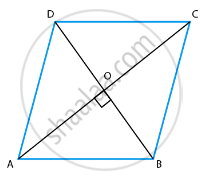# The lengths of the diagonals of a rhombus are 16 cm and 12 cm. Then, the length of the side of the rhombus is ______. - Mathematics

MCQ
Fill in the Blanks

The lengths of the diagonals of a rhombus are 16 cm and 12 cm. Then, the length of the side of the rhombus is ______.

• 9 cm

• 10 cm

• 8 cm

• 20 cm

#### Solution

The lengths of the diagonals of a rhombus are 16 cm and 12 cm. Then, the length of the side of the rhombus is 10 cm.

Explanation:

We know that,

A rhombus is a simple quadrilateral whose four sides are of same length and diagonals are perpendicular bisector of each other.

We get,AC = 16 cm and BD = 12 cm

∠AOB = 90°

∵ AC and BD bisects each other

AO = 1/2 AC and BO = 1/2 BD

Then we get,

AO = 8 cm and BO = 6 cm

Now, In right angled ∆AOB

Using the Pythagoras theorem,

We have,

AB2 = AO2 + OB2

AB2 = 82 + 62

= 64 + 36

= 100

∴ AB = sqrt(100) = 10 cm

We know that the four sides of a rhombus are equal.

Therefore, we get,

One side of rhombus = 10 cm.

Concept: Application of Pythagoras Theorem in Acute Angle and Obtuse Angle
Is there an error in this question or solution?

#### APPEARS IN

NCERT Mathematics Exemplar Class 10
Chapter 6 Triangles
Exercise 6.1 | Q 2 | Page 61

Share